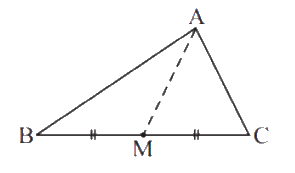# Ex.6.4 Q3 The-Triangle-and-its-Properties Solutions-NCERT Maths Class 7

Go back to  'Ex.6.4'

## Question

$$AM$$ is a median of a triangle $$ABC.$$ Is $$AB + BC + CA > 2 AM?$$ (Consider the sides of triangles $$\Delta{ABM}$$ and $$\Delta{AMC}$$.)Video Solution
Triangle & Its Properties
Ex 6.4 | Question 3

## Text Solution

What is known?

$$ABC$$ is a triangle and $$AM$$ is a median of triangle $$ABC$$.

What is unknown?

Is $$AB + BC + CA > 2\, AM?$$

Reasoning:

In this question it is asked if $$AB + BC + CA > 2 AM$$ or not. This question is also based on the property that the sum of lengths of two sides of a triangle is always greater than the third side. In such kind of problems, you just visually identify the triangle $$ABC$$ and $$AM$$ is the median which further divides triangle $$ABC$$ into two more triangles i.e. triangle $$ABM$$ and $$AMC.$$ Now consider any two sides of first triangle $$ABM$$ and use the above property then consider the any two sides of another triangle $$AMC$$ and use the above property. Now, add $$\rm{}L.H.S.$$and $$\rm{}R.H.S.$$ of both the triangles.

Steps:

In triangle $$ABM,$$

$$AB + BM > AM{\rm{ }} \qquad \ldots .{\rm{ }}\left( 1 \right)$$

In triangle $$AMC,$$

$$AC + MC > AM{\rm{ }} \qquad \ldots .{\rm{ }}\left( 2 \right)$$

Adding equation $$\rm{}(1)$$ and $$\rm{}(2)$$ we get,

\begin{align}&AB \!+\! BM \!+\! AC \!+\! MC \!>\! AM \!+\! AM\\&AB \!+\! AC \!+\! BM \!+\! MC \!>\! 2AM\\&AB \!+\! AC \!+\! BC \!>\! 2AM\end{align}

Hence, it is true

Useful Tip:

Whenever you encounter problems of this kind, it is best to think of the property based on sum of lengths of any two sides of a triangle is always greater than the third side

Learn from the best math teachers and top your exams

• Live one on one classroom and doubt clearing
• Practice worksheets in and after class for conceptual clarity
• Personalized curriculum to keep up with school